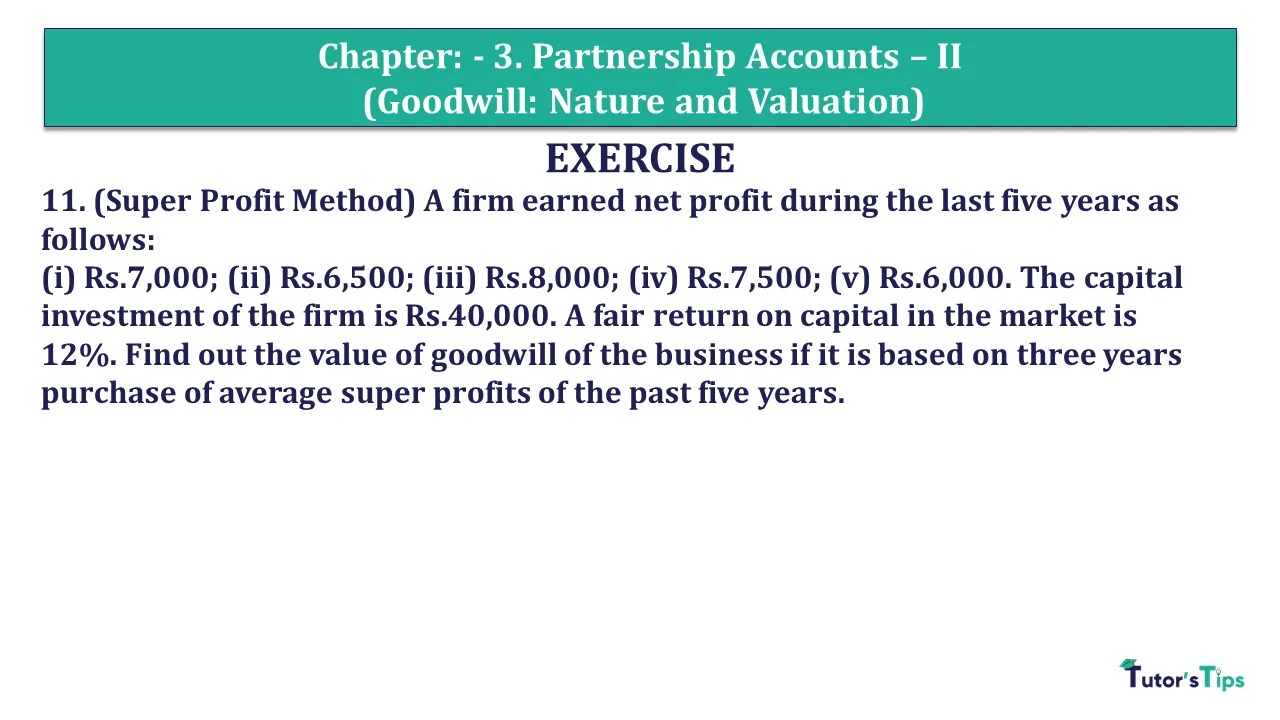# Question 11 Chapter 3 of +2 – USHA Publication 12 Class Part – 1Q-11 - CH-3 - Usha +2 Book 2018 - Solution

Question 11 Chapter 3 of +2- Usha

11. (Super Profit Method) A firm earned net profit during the last five years as follows:
(i) Rs.7,000; (ii) Rs.6,500; (iii) Rs.8,000; (iv) Rs.7,500; (v) Rs.6,000. The capital investment of the firm is Rs.40,000. A fair return on capital in the market is 12%. Find out the value of goodwill of the business if it is based on three years purchase of average super-profits of the past five years.

## The solution of Question 11 Chapter 3 of +2- Usha: –

### Formula to calculate the Value of Goodwill with Super Profit:

 Goodwill = Super Profit X Number of years’ purchase

Numbers of years’ of Purchase is given So firstly we have to calculate super profit with the help of following formula:

 Super Profit = Actual Average Profit – Normal Profit

To calcuate super profit we have to calculate the Actual Average profit and Normal Profit as following:

### Calculation of Average Profit: –

 Average Profit = Total Profit for past given years Number of years = 7,000 + 6,500 + 8,000 + 7,500 + 6,000 5 = 35,000 5 Average Profit = 7,000

### Calculation of Normal Profit: –

 Normal Profit = Capital Employed X Normal Rate of Return 100 = 40,000 X 12 100 Normal Profit = 4,800

### Calculation of Super Profit:

 Super Profit = Actual Average Profit – Normal Profit = 7,000 – 4,800 Super Profit = 2,200
 Number of years’ purchase = 3 (Given)

### Calculation of Value of Goodwill with Super Profit:

 Goodwill = Super Profit X Number of years’ purchase = 2,200 X 3 Goodwill = 6,600